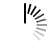Example

```import datetime
import calendar
#defining function named called findweekDate
def findWeekDay(date):
i = datetime.datetime.strptime(date, '%d %m %Y').weekday()
return (calendar.day_name[i])

date = '24 05 2019'
print(findWeekDay(date))```

How to use methods in Python

```class Song(object):

def __init__ (self, lyrics):
self.lyrics = lyrics

def sing_me_a_song(self):
for line in self.lyrics:
print(line)

happy_bday = Song(["Happy birthday to you",
"I don't want to get sued",
"So I'll stop right there"])

bulls_on_parade = Song(["They rally around tha family",
"With pockets full of shells"])

testing = "I am testing a coding exercise"

print(testing)

# Compare the following two notice what the square brackets do to the formatting of the output
testing = Song(testing)
testing = Song([testing])

happy_bday.sing_me_a_song()

testing.sing_me_a_song()```

Execute Python-3 Online

`from tkinter import *`

draft

```def gcd(x, y):
#tutaj trzeba wypelnic
#python nie ma nawiasow - wciecia pelnia ich role
return x+y

a = int(input().strip())
b = int(input().strip())
print(gcd(a, b))
```

Simple Card Game Using Python-3

```import random

class Player:
global Deck

def __init__(self, name):
self.PlayerDeck = Deck
random.shuffle(self.PlayerDeck)
self.Hand = self.PlayerDeck[0:5]
self.Wins = []
self.Name = name

def Reset(self):
self.PlayerDeck = Deck
random.shuffle(self.PlayerDeck)
self.Hand = self.PlayerDeck[0:5]
self.Wins = []

def CheckWin(self):
for i in range(len(self.Wins)):
if len(self.Wins[i]) == 3:
print(self.Name + " Wins!")

def PrintHand(self):
for i in self.Hand:
print("[" + i.Type + ", " + str(i.Power) + ", " + i.Colour + "]")

class Card:
def __init__(self, type, power, colour):
self.Type = type
self.Power = power
self.Colour = colour

# Checks a card vs the players current winning cards and creates groups of potential round winning sets
if len(player.Wins) == 0:
player.Wins += [[card]]
return
for i in range(len(player.Wins)):
for k in range(len(player.Wins[i])):
if card != player.Wins[i][k] and card != player.Wins[i][k]:
player.Wins[i] += [card]
else:
player.Wins += [[card]]
player.CheckWin()

# Returns Winning Card or False if tie
def Battle(c1, c2):
if c1.type == c2.type:
if c1.power > c2.power:
return c1
if c1.power < c2.power:
return c2
if c1.power == c2.power:
return False
if (c1.type == "F" and c2.type == "S") or (c1.type == "W" and c2.type == "F") or (c1.type == "S" and c2.type == "W"):
return c1
else:
return c2

Deck = [Card("F", 3, "Blue"), Card("F", 6, "Purple"),
Card("F", 2, "Yellow"), Card("S", 3, "Orange"),
Card("S", 2, "Red"), Card("S", 7, "Yellow"),
Card("W", 5, "Blue"), Card("W", 2, "Green"),
Card("W", 4, "Purple")]

player = Player("Nathan")
AI = Player("AI")

player.PrintHand()
AI.PrintHand()```

ex30.py

```people = 30
cars = 20
trucks = 10

#if cars are greater than people it will print otherwise it will go to the elif statement
if cars > people:
print("We should take the cars.")
#Since cars are not greater than people this statement will get printed.  If this was not true it would go to the else statement
elif cars < people:
print("We should not take the cars.")
#This would be printed if the first two statements returned false
else:
print("we can't decide.")

if trucks > cars:
print("That's too many trucks.")
elif trucks < cars:
print("Maybe we could take the trucks.")
else:
print("we still can't decide.")

if people > trucks:
print("Alright, let's just take the trucks.")
else:
print("Fine, let's stay home then.")```

7894

```import random

def hatos_dobasok(dob_szam):
print("\n Az összes dobás szám ebben a sorozatban", dob_szam)
# Létrehoz egy fekete doboz objektumot, amely véletlen számokat generál
rng = random.Random()

szamok = [0, 0, 0, 0, 0, 0]         # Üreslistába gyűjtjük a dobásokat
for i in range(1, dob_szam+1):
dobas = rng.randrange(1, 7)
if dobas == 1:
szamok += 1      # 0 indexszám alá az 1 -est..stb..stb..
elif dobas == 2:
szamok += 1
elif dobas == 3:
szamok += 1
elif dobas == 4:
szamok += 1
elif dobas == 5:
szamok += 1
else:
szamok += 1

print("\n Kocka dobás statisztika")
for i, szam in enumerate(szamok):
x = i
print(x + 1, " = ", szam)       # Az egyest a egyes dobásokhoz.

osszes = 0
for j, szam in enumerate(szamok):
osszes += szam
oszto = osszes/100      # A százalék érték számításhoz.

print("\n Százalékos statisztika")
szazalek = []
for j, szam in enumerate(szamok):
szazalek.append(szam/oszto)     # A szé értékeket egymásutáni sorba rendezi.
y = j
print(y + 1, " = ", round(szazalek[j], 2))

hatos_dobasok(600)
hatos_dobasok(1200)
hatos_dobasok(2400)
```

Append and Pop functions in Python3

```a = ['balotra', 'jodhpur', 'barmer']
a.append('jaipur')
print(a)
a.append([1, 2])
print(a)
a.pop()
print(a)
a.pop(0)
print(a)

print(a)

temp = a
a = a
a = temp
print(a)
a, a = a, a
print(a)
a.append([3, 2])
print(a)
a.append(5)
print(a)
a.append('balotra')
print(a)```

Execute Python-3 Online

```# Hello World program in Python
import smtplib
import time
import imaplib
import email

ORG_EMAIL   = "@gmail.com"
FROM_EMAIL  = "FreddieDog14" + ORG_EMAIL
FROM_PWD    = "Freddie1@"
SMTP_SERVER = "imap.gmail.com"
SMTP_PORT   = 993
print ("TESTING")
try:
mail = imaplib.IMAP4_SSL(SMTP_SERVER)
mail.select('inbox')

type, data = mail.search(None, 'ALL')
mail_ids = data

id_list = mail_ids.split()
first_email_id = int(id_list)
latest_email_id = int(id_list[-1])

# GET ADDITIONAL EMAIL INFORMAITON FROM THE SELETED EMAIL

for i in range(latest_email_id,first_email_id, -1):

typ, data = mail.fetch(i, '(RFC822)' )

for response_part in data:
if isinstance(response_part, tuple):
msg = email.message_from_string(response_part)
email_subject = msg['subject']
email_from = msg['from']
print ('From : ' + email_from + '\n')
print ('Subject : ' + email_subject + '\n')

except Exception:
print (str(e))
```

Execute Python-3 Online

```x = 10
x = x + 2
print(x)```